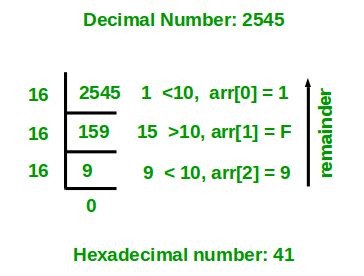Related Articles

# Program for decimal to hexadecimal conversion

• Difficulty Level : Easy
• Last Updated : 12 May, 2021

Given a decimal number as input, we need to write a program to convert the given decimal number into equivalent hexadecimal number. i.e convert the number with base value 10 to base value 16.

Hexadecimal numbers uses 16 values to represent a number. Numbers from 0-9 are expressed by digits 0-9 and 10-15 are represented by characters from A – F.

Examples:

```Input : 116
Output: 74

Input : 10
Output: A

Input : 33
Output: 21```

Algorithm:

1. Store the remainder when the number is divided by 16 in a temporary variable temp. If temp is less than 10, insert (48 + temp) in a character array otherwise if temp is greater than or equals to 10, insert (55 + temp) in the character array.
2. Divide the number by 16 now
3. Repeat the above two steps until the number is not equal to 0.
4. Print the array in reverse order now.

Example

If the given decimal number is 2545.

Step 1: Calculate remainder when 2545 is divided by 16 is 1. Therefore, temp = 1. As temp is less than 10. So, arr = 48 + 1 = 49 = ‘1’.
Step 2: Divide 2545 by 16. New number is 2545/16 = 159.
Step 3: Calculate remainder when 159 is divided by 16 is 15. Therefore, temp = 15. As temp is greater than 10. So, arr = 55 + 15 = 70 = ‘F’.
Step 4: Divide 159 by 16. New number is 159/16 = 9.
Step 5: Calculate remainder when 9 is divided by 16 is 9. Therefore, temp = 9. As temp is less than 10. So, arr = 48 + 9 = 57 = ‘9’.
Step 6: Divide 9 by 16. New number is 9/16 = 0.
Step 7: Since number becomes = 0. Stop repeating steps and print the array in reverse order. Therefore, the equivalent hexadecimal number is 9F1.

Below diagram shows an example of converting the decimal number 2545 to an equivalent hexadecimal number.Below is the implementation of above idea.

## C++

 `// C++ program to convert a decimal``// number to hexadecimal number` `#include ``using` `namespace` `std;` `// function to convert decimal to hexadecimal``void` `decToHexa(``int` `n)``{``    ``// char array to store hexadecimal number``    ``char` `hexaDeciNum;` `    ``// counter for hexadecimal number array``    ``int` `i = 0;``    ``while` `(n != 0) {``        ``// temporary variable to store remainder``        ``int` `temp = 0;` `        ``// storing remainder in temp variable.``        ``temp = n % 16;` `        ``// check if temp < 10``        ``if` `(temp < 10) {``            ``hexaDeciNum[i] = temp + 48;``            ``i++;``        ``}``        ``else` `{``            ``hexaDeciNum[i] = temp + 55;``            ``i++;``        ``}` `        ``n = n / 16;``    ``}` `    ``// printing hexadecimal number array in reverse order``    ``for` `(``int` `j = i - 1; j >= 0; j--)``        ``cout << hexaDeciNum[j];``}` `// Driver program to test above function``int` `main()``{``    ``int` `n = 2545;` `    ``decToHexa(n);` `    ``return` `0;``}`

## Java

 `// Java program to convert a decimal``// number to hexadecimal number``import` `java.io.*;` `class` `GFG {``    ``// function to convert decimal to hexadecimal``    ``static` `void` `decToHexa(``int` `n)``    ``{``        ``// char array to store hexadecimal number``        ``char``[] hexaDeciNum = ``new` `char``[``100``];` `        ``// counter for hexadecimal number array``        ``int` `i = ``0``;``        ``while` `(n != ``0``) {``            ``// temporary variable to store remainder``            ``int` `temp = ``0``;` `            ``// storing remainder in temp variable.``            ``temp = n % ``16``;` `            ``// check if temp < 10``            ``if` `(temp < ``10``) {``                ``hexaDeciNum[i] = (``char``)(temp + ``48``);``                ``i++;``            ``}``            ``else` `{``                ``hexaDeciNum[i] = (``char``)(temp + ``55``);``                ``i++;``            ``}` `            ``n = n / ``16``;``        ``}` `        ``// printing hexadecimal number array in reverse``        ``// order``        ``for` `(``int` `j = i - ``1``; j >= ``0``; j--)``            ``System.out.print(hexaDeciNum[j]);``    ``}` `    ``// driver program``    ``public` `static` `void` `main(String[] args)``    ``{``        ``int` `n = ``2545``;``        ``decToHexa(n);``    ``}``}` `// Contributed by Pramod Kumar`

## Python3

 `# Python3 program to``# convert a decimal``# number to hexadecimal``# number` `# function to convert``# decimal to hexadecimal`  `def` `decToHexa(n):` `    ``# char array to store``    ``# hexadecimal number``    ``hexaDeciNum ``=` `[``'0'``] ``*` `100` `    ``# counter for hexadecimal``    ``# number array``    ``i ``=` `0``    ``while``(n !``=` `0``):` `        ``# temporary variable``        ``# to store remainder``        ``temp ``=` `0` `        ``# storing remainder``        ``# in temp variable.``        ``temp ``=` `n ``%` `16` `        ``# check if temp < 10``        ``if``(temp < ``10``):``            ``hexaDeciNum[i] ``=` `chr``(temp ``+` `48``)``            ``i ``=` `i ``+` `1``        ``else``:``            ``hexaDeciNum[i] ``=` `chr``(temp ``+` `55``)``            ``i ``=` `i ``+` `1``        ``n ``=` `int``(n ``/` `16``)` `    ``# printing hexadecimal number``    ``# array in reverse order``    ``j ``=` `i ``-` `1``    ``while``(j >``=` `0``):``        ``print``((hexaDeciNum[j]), end``=``"")``        ``j ``=` `j ``-` `1`  `# Driver Code``n ``=` `2545``decToHexa(n)` `# This code is contributed``# by mits.`

## C#

 `// C# program to convert a decimal``// number to hexadecimal number``using` `System;` `class` `GFG {``    ``// function to convert decimal``    ``// to hexadecimal``    ``static` `void` `decToHexa(``int` `n)``    ``{``        ``// char array to store``        ``// hexadecimal number``        ``char``[] hexaDeciNum = ``new` `char``;` `        ``// counter for hexadecimal number array``        ``int` `i = 0;``        ``while` `(n != 0) {``            ``// temporary variable to``            ``// store remainder``            ``int` `temp = 0;` `            ``// storing remainder in temp``            ``// variable.``            ``temp = n % 16;` `            ``// check if temp < 10``            ``if` `(temp < 10) {``                ``hexaDeciNum[i] = (``char``)(temp + 48);``                ``i++;``            ``}``            ``else` `{``                ``hexaDeciNum[i] = (``char``)(temp + 55);``                ``i++;``            ``}` `            ``n = n / 16;``        ``}` `        ``// printing hexadecimal number``        ``// array in reverse order``        ``for` `(``int` `j = i - 1; j >= 0; j--)``            ``Console.Write(hexaDeciNum[j]);``    ``}` `    ``// Driver Code``    ``public` `static` `void` `Main(String[] args)``    ``{``        ``int` `n = 2545;``        ``decToHexa(n);``    ``}``}` `// This code is contributed by Nitin Mittal.`

## PHP

 `= 0; ``\$j``--)``        ``echo` `\$hexaDeciNum``[``\$j``];``}` `// Driver Code``\$n` `= 2545;``decToHexa(``\$n``);` `// This code is contributed``// by mits.``?>`

## Javascript

 ``
Output
`9F1`

Using Predefined function

## Java

 `// Java program to convert a decimal``// number to hexadecimal number``import` `java.io.*;` `class` `GFG {``    ``public` `static` `void` `decToHexa(``int` `n)``    ``{``        ``System.out.println(Integer.toHexString(n));``    ``}``    ``public` `static` `void` `main(String[] args)``    ``{` `        ``int` `n = ``2545``;``        ``decToHexa(n);``    ``}``}`
Output
`9f1`

This article is contributed by Harsh Agarwal. If you like GeeksforGeeks and would like to contribute, you can also write an article using write.geeksforgeeks.org or mail your article to contribute@geeksforgeeks.org. See your article appearing on the GeeksforGeeks main page and help other Geeks.